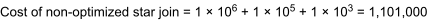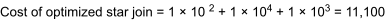# 17.10 - Star and Snowflake Join Optimization - Advanced SQL Engine - Teradata Database

## Teradata Vantage™ - SQL Request and Transaction Processing

Product
Release Number
17.10
Release Date
July 2021
Content Type
Programming Reference
User Guide
Publication ID
B035-1142-171K
Language
English (United States)

## About Star and Snowflake Join Optimizations

Star and snowflake joins are terms used to describe various large table/small table joins. The following concepts apply when optimizing star and snowflake joins:
• Large and small are relative terms.

Generally the ratio of the cardinalities of the large table to each of the small tables ranges from 100:1 to 1,000:1.

Note that these cardinality ratios apply to the results tables being joined, not to the base tables before they are reduced by predicate qualifications.

• The join plan in which all small tables are joined first is called the product/merge join plan because the small tables are usually joined via a product join and the joined result of the small tables is usually joined to the large table via a merge join.
The EXPLAIN text for queries involving star/snowflake or LT/ST joins does not explicitly mention them.

## Star Joins

A star join schema is one in which a large table, called a fact table, is joined to a set of smaller tables, called dimension tables.

The fact table has a composite primary index. Each dimension table in a set related to a fact table has a simple primary index that corresponds exactly to one of the components of the composite primary index in the fact table.

Star join queries do not place selection criteria directly on a dimension table. Rather they place an IN condition on the primary index of the dimension table that is stored in the fact table. The IN list behaves as if it were a dimension table, thus allowing star join processing to occur in cases where normally the dimension table would have been required.

The Optimizer can apply star join processing to requests that join a subset of primary index/NUSI columns of a fact table to dimension tables and qualify the remaining primary index/NUSI columns with IN conditions.

A star join is a join where three or more relations with relatively small cardinality are joined to another relation having a much larger relative cardinality. In dimensional modeling terminology, the large table is called a fact table and the smaller tables are referred to as dimension tables.

The difference between a large relation and a small relation is defined in terms of cardinality ratios on the order of 100:1 at minimum. The smaller relations in a star join do not necessarily derive from base tables having a smaller cardinality; they can be an intermediate result of having applied a condition to a medium- or large-sized base table.

The term star derives from the pictorial representation of such a join, which resembles a childlike drawing of a star, as seen in the following illustration.

As you can see, the graphic representation of such a join resembles a crude drawing of a star.## Primary and Secondary Targets of a Star Join

Star joins are a fundamental component of a dimensional database model. Therefore, the primary target of star join processing is the product join of numerous small relations to build a composite column set that can be used to access a large relation directly as either its primary index or a secondary index.

The star join method is useful in the following situations:
• Complex cases involving multiple large relations.
• A product join of several small relations in order to permit a merge join or a hash join with a locally spooled large relation when there is no available index.

## Snowflake Joins

A snowflake join is a join where a large table is joined with three or more smaller base tables or join relations, some or all of which themselves are joined to three or more smaller base tables or join relations. Another way of looking at a snowflake is to think of it as a star with normalized dimension tables.

The concept of snowflake schemas in physical database design is described in Teradata Vantage™ - Database Design, B035-1094.

As with a star join, the cardinality ratios determine whether a table or join relation is large or small. The minimum cardinality ratio defining a large:small table relationship is 100:1.

The graphic representation of such a join somewhat resembles a snowflake.The concept of snowflake schemas in physical database design is described in Teradata Vantage™ - Database Design, B035-1094.

## Star Join Optimization

With star join optimization, the Optimizer searches for a better join plan in which all the small tables are joined first, after which the resulting relation is joined with the large table. The Optimizer then uses the join plan that has the lowest estimated cost.

Without star join optimization, the Optimizer does an adequate job joining one or two small tables to a large table. However, when joining three or more small tables to one large table, the Optimizer usually generates a join plan in which a small table (or the join result of small tables) is joined directly with the large table.

When one or more IN conditions are specified on the large table, the Optimizer might choose to combine the IN lists with the small tables first. The query plan would then join the resulting join relation with the large table. The result is a star join plan with more dimension tables than the number of dimension tables explicitly specified in the query (see stage 6 in Determining the Order of Joins).

## Example of Star Join Optimization

The following graphic illustrates a non-optimal star join plan of four tables. The relative cardinalities of the tables are given as integers within each table (represented in each case by a circle). The relative cost of each join is given as an integer number on the line connecting the joined relations.

In this example, the first join is between the large table and one of the small tables. The relative cost of this join is 1 x 106. The next join is between this joined relation and another of the small tables. Its relative cost is 1 x 105. Finally, this relation is joined with the last small table at a relative cost of 1 x 103.The next graphic presents an optimized join plan for the same set of tables. This plan uses a compromise join of all the unconnected (small) tables prior to making the join to the large table.

The first join has a relative cost of 1 x 102, the second a cost of 1 x 104, and the final join between the large table and its joined small tables relation has a cost of 1 x 103.The results indicate that for this example, the optimized star join plan is 2 orders of magnitude cheaper than the non-optimized star join plan for the same 4 tables.

## Star Join Categories

Star joins are also referred to as large-table/small-table (LT/ST) joins. There are two basic types of LT/ST joins: